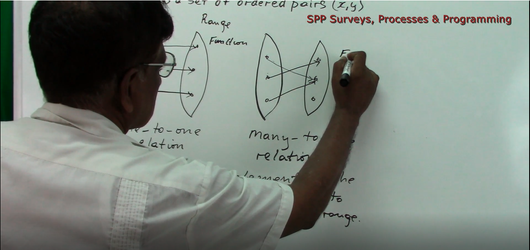# Functions and Relations - Introduction

Regular price \$2.00 Sale

Introduction to Functions and Relations. This 17:08 minute (800MB) Math Tutorial is conducted by Mr. Chan (Trained Certified Teacher).

Topics covered in this tutorial are:

• One to One Relation
• Many to One Relation
• Notations for Functions (f:x, f(x), x – Y Notation)
• Representing Relations
• Ordered Pairs
• Graph
• Table
• Mapping Diagram
• Linear Functions